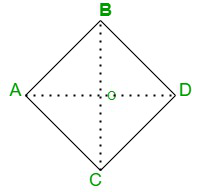# How to find the area of a Rhombus?

• Last Updated : 29 Oct, 2021

Mensuration is a part of geometry that deals with the study or measurements of the area, perimeter, and volume of two-dimensional or three-dimensional shapes and structures. Mensuration includes basic mathematical formulas and even algebraic expressions in some cases.

The below-given article is also a study of mensuration. It explains the two-dimensional structure of the rhombus and its area formulas. All three ways of computing an area of a rhombus are mentioned in the article.

What is Rhombus?

Rhombus is a two-dimensional geometrical shape having four sides that are equal in length and are congruent.### How to find the area of a Rhombus?

The area of a rhombus is the total space covered or enclosed by the rhombus on a two-dimensional plane. The area of the rhombus can be calculated by three different methods by using diagonal, using base and height, and using trigonometry.

Area formula

• Using diagonal

Area = (d1 × d2)/2 sq. units

Where,

d1 is the length of diagonal 1

d2  is the length of diagonal 2

• Using Base and height

Area of a Rhombus = base × height sq units

Where,

b is the length of any side of the rhombus

h is the height of the rhombus

• Using Trigonometry

Area of a Rhombus = b2 × sin(A) sq. units

Where,

b is the length of any side of the rhombus

A is a measure of any interior angle

### Derivation of area formula of Rhombus

Let us consider a rhombus ABCD with O as the point of intersection of two diagonals AC and BD.The area of rhombus will be

Area = 4 × area of △AOB

= 4 × (1/2) × AO × OB sq.units

= 4 × (1/2) × (1/2) d1 × (1/2) d2 sq.unit

= 4 × (1/8) d1 × d2

= 1/2 d1 × d2

Therefore, the area of a rhombus is A = 1/2 d1 × d2.

### Sample Questions

Question 1. Calculate the area of a rhombus (using base and height) if its base is 5cm and height is 3cm.

Solution:

Given,

Base (b) = 5cm

height of rhombus(h) = 3cm

Now,’

Area of the rhombus(A) = b × h

= 5 × 3

= 15cm2

Question 2. Calculate the area of a rhombus (using diagonal) having diagonals equal to 4cm and 3cm.

Solution:

Given,

Length of diagonal 1 (d1) = 4cm

Length of diagonal 2 (d2) = 3cm

Now,

Area of Rhombus (A) = 1/2 d1 × d2

= 4 x3/2 = 6cm2

Question 3. Calculate the area of the rhombus (using trigonometry) if its side is 8cm and one of its angles A is 30 degrees.

Solution:

Side of the rhombus (b) = 8cm

angle (a) = 30 degrees

Now,

Area of the rhombus(A) = b2 × sin(a)

= (8) × sin(30)

= 64 × 1/2 = 32 cm2

Question 4. How to calculate the perimeter of a rhombus?

Solution:

The perimeter of a rhombus can be calculated by the formula

P= 4b units

where b is a side of the rhombus.

My Personal Notes arrow_drop_up